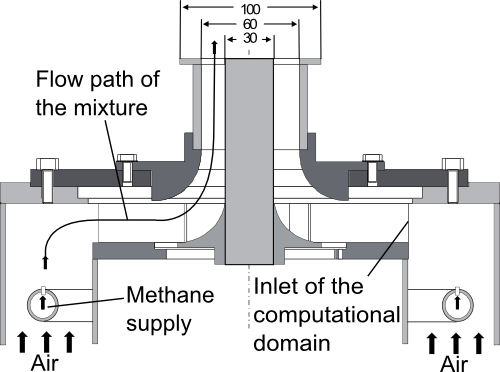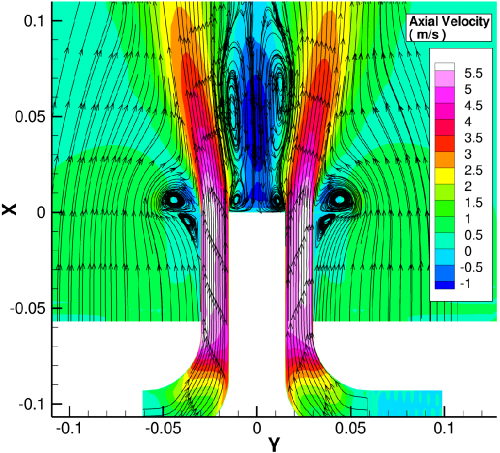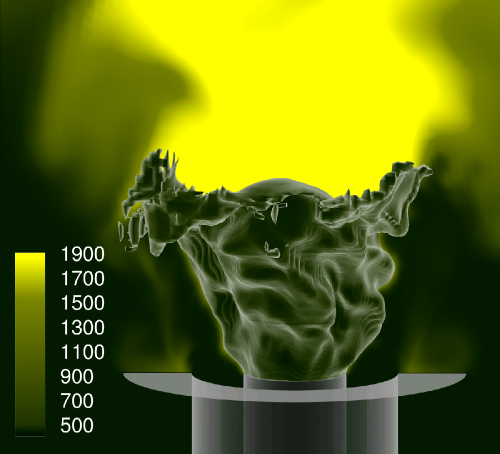# Premixed Methane-Air Swirl Burner (TECFLAM)

## Introduction

The TECFLAM swirl burner is designed to investigate essential features found in industrial lean premixed combustors. An extensive set of measurements obtained by advanced laser-diagnostics exists to:

• Give insight into the underlying physics to gain a deeper understanding of the relevant phenomena
• Build a database well suited for CFD validation

Validation data exists for the reacting and non-reacting cases using the same velocity boundary conditions to allow for a separate assessment of the applied turbulence model and combustion model.

Even though the swirl nozzle design is similar to the case AC2-06 there are fundamental differences regarding the resulting flame structure. Contrary to the diffusion burner presented in AC2-06, which is confined in a combustor, the present configuration features an unconfined lean premixed flame. Compared to the AC2-06 diffusion burner, which has 2 annuli with the inner one exiting methane and the outer one air, the methane supply through the inner annulus has been replaced by a water-cooled bluff body. The methane is now injected through a perforated ring line further upstream (see Fig.2.1) to obtain a homogeneous mixture before the reaction takes place. Hence, a flammable mixture exists before entering the reaction zone which yields major differences in the resulting temperature and species distribution and hence the modeling approach for the simulation needs to be chosen accordingly.

## Relevance to Industrial Sector

This test case features some important properties which can already be found in current combustors and will become of increasing importance in the future. These are:

• Swirl stabilization
• Premixed flame
• Lean combustion

The swirl stabilization is common practice in gas turbine combustors which allows the design of a very compact premixed flame with a high power density. Regarding the pollutant formation (such as the emission of nitric oxides, NOx) lean premixed combustion is of increasing importance in many industrial applications (such as land based gas turbines, aero-engines and automotive engines) due to the lower peak temperature when compared to non-premixed systems. Contrary to industrial applications this burner operates under atmospheric conditions (temperature = 300 K, pressure = 101325 Pa).

## Design or Assessment Parameters

The parameters to validate CFD simulations are radial profiles at different axial positions downstream from the nozzle exit. These are:

• Velocity
• Temperature
• Major species

Furthermore the frequency spectrum based on the autocovariance has been measured to identify the precessing vortex core (PVC), a large coherent structure present under isothermal conditions.

## Flow Geometry

The nozzle consists of a 15-mm wide annular slot surrounding a 30-mm diameter, water-cooled bluff-body. The bluff-body temperature was stabilized at 353 K to avoid water condensation. Swirl was generated by a movable block. The nozzle was placed concentrically inside a co-axial airflow 600 mm in diameter. As illustrated in Fig. 2.1, the air enters the configuration from the bottom where methane is injected using a perforated ring line. Then the methane-air mixture is deflected by 90 degree to enter the radial and tangential channels (see Fig. 2.2) where the swirl is generated. Hereafter the fuel moves upward again, passes the annulus around the bluff-body and enters the unconfined section. To achieve defined boundary conditions, the burner was placed into a co-axial air flow, emanating with 0.5m/s.Figure 2.1: Geometry of the Tecflam bluff-body swirl nozzle. 2-D cut of the experimental setup.

## Flow Physics

The main flow parameters are:

• The bulk velocity of the methane-air jet issuing from the annulus around the bluff-body: $u_{b}\approx 5\,\mathrm {m/s}$(case 30 KW), $u_{b}\approx 21\,\mathrm {m/s}$(case 150 kW), (Ma << 1)
• The Reynolds number based on the bulk velocity and the hydraulic diameter ($D_{h}=30\,\mathrm {mm}$): $Re\approx 10,000$(case 30 kW) , $Re\approx 42,000$(case 150 kW)
• The swirl number which represents the ratio of azimuthal and axial momentum at the nozzle exit: $S={\frac {\int _{R_{i}}^{R_{a}}{\overline {u}}{\overline {w}}+{\overline {u'w'}})r^{2}dr}{D_{h}\int _{R_{i}}^{R_{a}}({\overline {u^{2}}}+{\overline {u^{,2}}})rdr}}\approx 0.7$, where $R_{i}$and $R_{a}$denote the inner and outer radius of the annulus respectively
• The flame, which stabilizes by the recirculation of hot gases above the bluff-body, has a thermal power of 30 kW and 150 kW respectively and covers the range of 1 < Ka < 4 and 4 < Da < 20 (Ka= Karlovitz number, Da=Damkoehler number). Herein the Karlovitz number denotes the ratio of the flame thickness to the Kolmogorov length and the Damkoehler number corresponds to the ratio of the integral time scale to the chemical time scale. The values obtained for this flame indicate that the scales which characterize the turbulent flow field and the combustion chemistry are of the same order of magnitude. As given in the 'Test Data' section this leads to the regime diagram classification of a thickened wrinkled flame.
• The equivalence ratio of the methane-air mixture: $\phi =0.83$(case 30 kW), $\phi =1.0$(case 150 kW)
• The coflow velocity of $u=0.5$m/s (see also Fig. 2.4)

To provide an impression of the flow field Fig. 2.4 shows time averaged streamlines of the isothermal case to illustrate the expansion of the velocity field right after the nozzle exit and the formation of the central recirculation zone above the bluff-body. To give an illustration of the flame stabilization in the shear layer above the bluff-body and the intense flame turbulence interaction a snapshot of the reacting case simulation is shown in Fig. 2.5.Figure 2.4: Illustration of the flow field in the isothermal case. Streamlines and contour of axial velocity obtained from the simulation.Figure 2.5: Illustration of instantaneous flame turbulence interaction. Isosurface of the chemical source term and a slice extracted at z = 0mm showing the temperature field ( K ) .